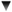ArticleS. UncleBob.

### Is a List of Circles a List of Shapes?

Given the following:
`  public interface Shape {    void draw();  }  public class Circle implements Shape {    public void draw() {...}  }`

Can you assign a List<Circle> to a List<Shape>? In other words, is a list of circles a subtype of a list of shapes?

With the advent of generics to Java, this old C++ issue comes to life once more for Java. And the architects of the Java language have added some clever, if perhaps opaque features to the language to deal with this issue.

So, is the following legal?
`  void f(List<Shape> ls) {/*...*/ }  void g() {    List<Circle> lc = new ArrayList<Circle>();    /*...*/    f(lc);  } `

 Error: f(java.util.List) cannot be applied to (java.util.List)

So clearly the compiler does not think that a List<Circle> ISA List<Shape>. That's interesting since a Circle ISA Shape. So apparently a list of derivatives is not a derivative of a list of bases. But why?

The answer is easy to understand. Let's say that the code above was valid. then I could implement f as follows:
`  void f(List<Shape> ls) {    ls.add(new Square());  } `

That would be bad! We don't want to be able to put a Square into a list of Circles. And yet you can put a Square into a List<Shape>. This makes it obvious that a List<Circle> cannot be substituted for a List<Shape>.

But wait. What if all we wanted to do in f is to do things that would be safe. For example what if f were implemented as follows:
`  void f(List<Shape> ls) {    for (Shape s : ls)      s.draw();  }`

Clearly it would be perfectly safe to pass a List<Circle> to this function. So it's too bad that the compiler doesn't allow it.

But wait! The compiler does allow it. We just have to use a different syntax. Look carefully at the following:
`  void f(List<? extends Shape> ls) {    for (Shape s : ls)      s.draw();  }  void g() {    List<Circle> lc = new ArrayList<Circle>();    /*...*/    f(lc);  }`

This compiles!

The List<? extends Shape> specifies a list of objects who's types are unknown (that's what the ? means) but whose types are derived from or equal to Shape.

So this solves the problem. We can pass List<Circle> to List<? extends Shape>. Does this mean that List<Circle> ISA List<? extends Shape>? Well, not quite. You see, in order to allow us to pass List<Circle> to functions that take a List<? extends Shape>, the compiler has to make certain operations on List<? extends Shape> illegal. Specifically, it must make it illegal to add to the list. Consider this:
`  void f(List<? extends Shape> ls) {    ls.add(new Circle());  }`

The compiler complains about this with the same error as before. You are not allowed to add Circles to lists of things that derive from shape. As odd as this sounds, it is necessary to prevent us from adding a Square to a List<Circle> that was passed into f. Another way to look at this is that List<? extends Shape> is a list of objects who's type is unknown. And that means that we can't add objects to it, because we don't know what type of objects to add.

Is this useful? I suppose so. There were several times in C++ where I ran into situations where I wanted to pass lists of derivatives to functions that took lists of bases. But I didn't run accross it that often. So I'm ambivalent about this. It's extra syntax, and the syntax is odd. But I suppose it'll be useful in a few situations.

On the other hand List<? super Shape> is another story altogether. We'll talk about that in another blog.

!commentFormSun, 6 Nov 2005 05:20:46, Ronald Mai, the shape list has already known its element type
`  void f(List<? extends Shape> ls) {    ls.add(new Circle());  }`
`  void f(List<? extends Shape> ls) {    ls.appendANewElement();  }`Mon, 7 Nov 2005 04:57:01, Yuguo Gong, C++ solution wanted.Mon, 7 Nov 2005 19:08:32, Uncle Bob, Solution in C++
`template <class T> class ListDerivative : public List<T> {  public:    virtual void add(T t) {throw "can't add to ListDerivative";}}`